# 词嵌入：编码形式的词汇语义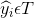• The mathematician ran to the store.

• The physicist ran to the store.

• The mathematician solved the open problem.

• The physicist solved the open problem.

• 我们发现数学家和物理学家在句子里有相同的作用，所以在某种程度上，他们有语义的联系。

• 当看见物理学家在新句子中的作用时，我们发现数学家也有起着相同的作用。

## 1. Getting Dense Word Embeddings（密集词嵌入）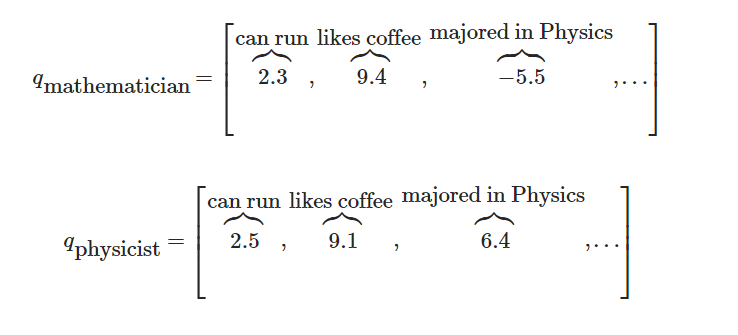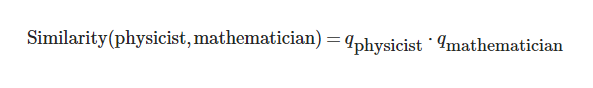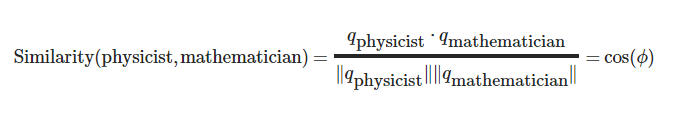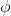是两个向量的夹角。这就意味着，完全相似的单词相似度为1。完全不相似的单词相似度为-1。

## 2. Pytorch中的词嵌入

``````# 作者: Robert Guthrie

import torch
import torch.nn as nn
import torch.nn.functional as F
import torch.optim as optim

torch.manual_seed(1)
``````
``````word_to_ix = {"hello": 0, "world": 1}
embeds = nn.Embedding(2, 5)  # 2 words in vocab, 5 dimensional embeddings
lookup_tensor = torch.tensor([word_to_ix["hello"]], dtype=torch.long)
hello_embed = embeds(lookup_tensor)
print(hello_embed)
``````
• 输出结果：
``````tensor([[ 0.6614,  0.2669,  0.0617,  0.6213, -0.4519]],
``````

## 3.例子： N-Gram语言模型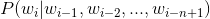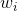是单词序列的第 i 个单词。 在本例中，我们将在训练样例上计算损失函数，并且用反向传播算法更新参数。

``````CONTEXT_SIZE = 2
EMBEDDING_DIM = 10
# 我们用莎士比亚的十四行诗 Sonnet 2
test_sentence = """When forty winters shall besiege thy brow,
And dig deep trenches in thy beauty's field,
Thy youth's proud livery so gazed on now,
Will be a totter'd weed of small worth held:
Then being asked, where all thy beauty lies,
Where all the treasure of thy lusty days;
To say, within thine own deep sunken eyes,
Were an all-eating shame, and thriftless praise.
How much more praise deserv'd thy beauty's use,
If thou couldst answer 'This fair child of mine
Shall sum my count, and make my old excuse,'
Proving his beauty by succession thine!
This were to be new made when thou art old,
And see thy blood warm when thou feel'st it cold.""".split()
# 应该对输入变量进行标记，但暂时忽略。
# 创建一系列的元组，每个元组都是([ word_i-2, word_i-1 ], target word)的形式。
trigrams = [([test_sentence[i], test_sentence[i + 1]], test_sentence[i + 2])
for i in range(len(test_sentence) - 2)]
# 输出前3行，先看下是什么样子。
print(trigrams[:3])

vocab = set(test_sentence)
word_to_ix = {word: i for i, word in enumerate(vocab)}

class NGramLanguageModeler(nn.Module):

def __init__(self, vocab_size, embedding_dim, context_size):
super(NGramLanguageModeler, self).__init__()
self.embeddings = nn.Embedding(vocab_size, embedding_dim)
self.linear1 = nn.Linear(context_size * embedding_dim, 128)
self.linear2 = nn.Linear(128, vocab_size)

def forward(self, inputs):
embeds = self.embeddings(inputs).view((1, -1))
out = F.relu(self.linear1(embeds))
out = self.linear2(out)
log_probs = F.log_softmax(out, dim=1)
return log_probs

losses = []
loss_function = nn.NLLLoss()
model = NGramLanguageModeler(len(vocab), EMBEDDING_DIM, CONTEXT_SIZE)
optimizer = optim.SGD(model.parameters(), lr=0.001)

for epoch in range(10):
total_loss = 0
for context, target in trigrams:

# 步骤 1\. 准备好进入模型的数据 (例如将单词转换成整数索引,并将其封装在变量中)
context_idxs = torch.tensor([word_to_ix[w] for w in context], dtype=torch.long)

# 步骤 2\. 回调torch累乘梯度
# 在传入一个新实例之前，需要把旧实例的梯度置零。

# 步骤 3\. 继续运行代码，得到单词的log概率值。
log_probs = model(context_idxs)

# 步骤 4\. 计算损失函数（再次注意，Torch需要将目标单词封装在变量里）。
loss = loss_function(log_probs, torch.tensor([word_to_ix[target]], dtype=torch.long))

# 步骤 5\. 反向传播更新梯度
loss.backward()
optimizer.step()

# 通过调tensor.item()得到单个Python数值。
total_loss += loss.item()
losses.append(total_loss)
print(losses)  # 用训练数据每次迭代，损失函数都会下降。
``````
• 输出结果：
``````[(['When', 'forty'], 'winters'), (['forty', 'winters'], 'shall'), (['winters', 'shall'], 'besiege')]
[523.1487259864807, 520.6150465011597, 518.0996162891388, 515.6003141403198, 513.1156675815582, 510.645352602005, 508.1888840198517, 505.74565410614014, 503.314866065979, 500.8949146270752]
``````

## 4.练习：计算连续词袋模型的词向量

CBOW 模型如下所示：给定一个单词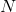代表两边的滑窗距，如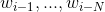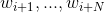， 并将所有的上下文词统称为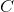，CBOW 试图最小化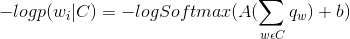• 考虑下你需要定义哪些参数。

• 确保你知道每步操作后的结构，如果想重构，请使用`.view()`

``````CONTEXT_SIZE = 2  # 左右各两个词
raw_text = """We are about to study the idea of a computational process.
Computational processes are abstract beings that inhabit computers.
As they evolve, processes manipulate other abstract things called data.
The evolution of a process is directed by a pattern of rules
called a program. People create programs to direct processes. In effect,
we conjure the spirits of the computer with our spells.""".split()

# 通过对`raw_text`使用set()函数，我们进行去重操作
vocab = set(raw_text)
vocab_size = len(vocab)

word_to_ix = {word: i for i, word in enumerate(vocab)}
data = []
for i in range(2, len(raw_text) - 2):
context = [raw_text[i - 2], raw_text[i - 1],
raw_text[i + 1], raw_text[i + 2]]
target = raw_text[i]
data.append((context, target))
print(data[:5])

class CBOW(nn.Module):

def __init__(self):
pass

def forward(self, inputs):
pass

# 创建模型并且训练。这里有些函数帮你在使用模块之前制作数据。

def make_context_vector(context, word_to_ix):
idxs = [word_to_ix[w] for w in context]
``````[(['We', 'are', 'to', 'study'], 'about'), (['are', 'about', 'study', 'the'], 'to'), (['about', 'to', 'the', 'idea'], 'study'), (['to', 'study', 'idea', 'of'], 'the'), (['study', 'the', 'of', 'a'], 'idea')]